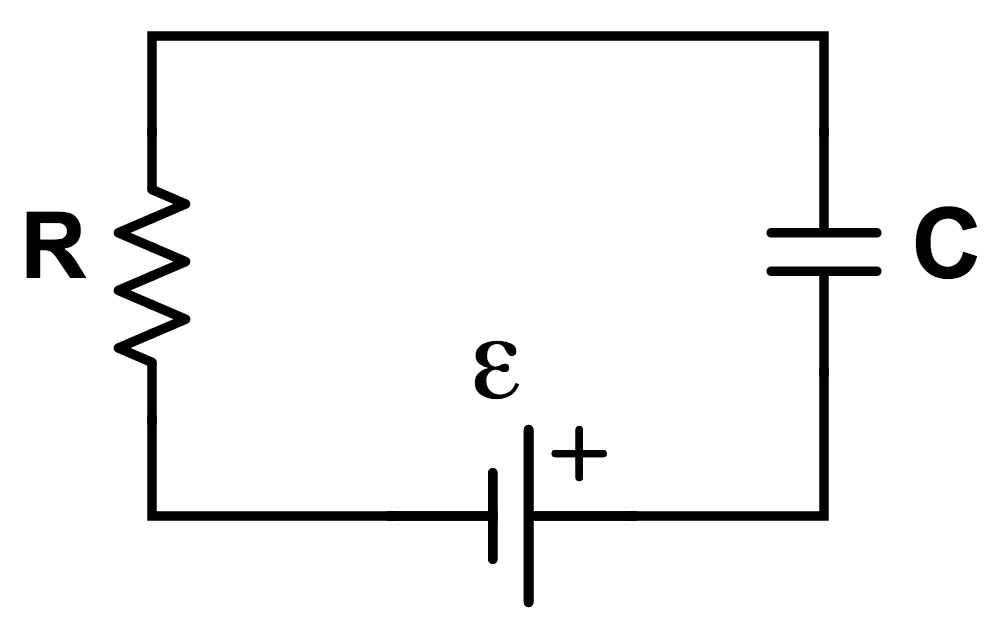# RC charging circuit

A 5 $\text{F}$ capacitor is connected in series to a 4 $\text{V}$ battery, a 3 $\Omega$ resistor, and a switch. What is the charge on the capacitor, in $\text{C}$, when the switch has been closed for $15 \ln10 \text{ s}$?×